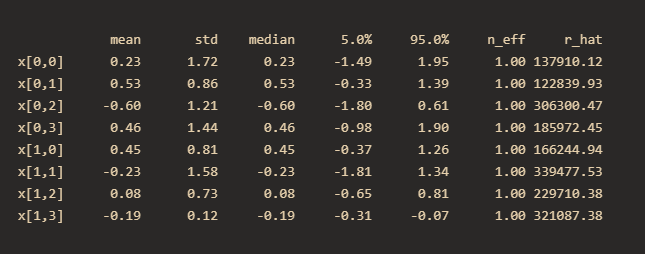# My observed variables are categorical, but they have different numbers of categories. How should I handle them?

Here’s some code I’ve written, but I’m struggling to make sense of how to fix it.
My observed variables consist of two categorical variables. The first variable ranges from [0,1,2], and the second variable ranges from [0,1,2,3]. Therefore, I multiplied logit_p by a specific matrix so that some elements in logit_p become 0. However, I found that the model did not converge after this operation. How can I achieve this goal correctly in numpyro? Are there any tools in numpyro that can facilitate this process?
My code is here:

``````def test():
# with numpyro.handlers.seed(rng_seed=1):
x = numpyro.sample("x", dist.Normal(0,2).expand([2,4]))

logit_p = jnp.exp(x)*jnp.array([[1,1,1,0],[1,1,1,1]])
p = logit_p/logit_p.sum(axis=-1).reshape(-1,1)
y = numpyro.sample("y",dist.Categorical(p), obs=jnp.array([0,1]))

kernel = NUTS(test)
mcmc = MCMC(kernel, num_warmup=2000, num_samples=2000,num_chains=2)
mcmc.run(random.PRNGKey(11))
mcmc.print_summary()
``````

And my output is here:Instead of masking, you can just simply let the model learn that prob of the category 3 for the first variable is 0

``````def test():
x = numpyro.sample("x", dist.Normal(0,2).expand([2,4]))
y = numpyro.sample("y", dist.Categorical(logits=x), obs=jnp.array([0, 1]))
``````
1 Like